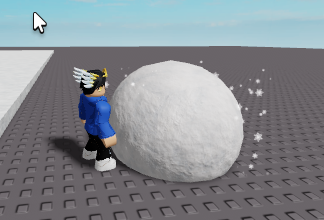# How would I make the snowball launch forward?

Hey Developers!, I have come with a question, how would i make the snowball launch forward when i press the F key?

I have already worked on the F key part but how do i make it launch forward?

Here is an image of what I’m talking aboutThanks!1 Like

You can set the snowball part’s network owner to the server and then use :ApplyImpulse at the player’s humanoid root part’s cframe lookvector multiplied by the mass of the ball. And if gravity is an issue you can try adding a -gravity/2 y vector to the lookvector.

You can directly set the velocity of something by setting the AssemblyLinearVelocity property. Alternatively you can apply an “instantaneous force” using the ApplyImpulse method.

Can you Provide a Code Example so i can see how to add this to my existing code?

``````local Ball = --your ball
local HumCF = HumanoidRootPart.CFrame --humanoid root parts cframe
local PowerMult = 10 --any number multiplier

Ball:SetNetworkOwner() --set to server so server can calculate physics
local vector = HumCF.LookVector * Ball.Mass * PowerMult + Vector3.new(0, workspace.Gravity/2, 0)
Ball:ApplyImpulse(vector)
``````

Alright I will give this a try and let you know